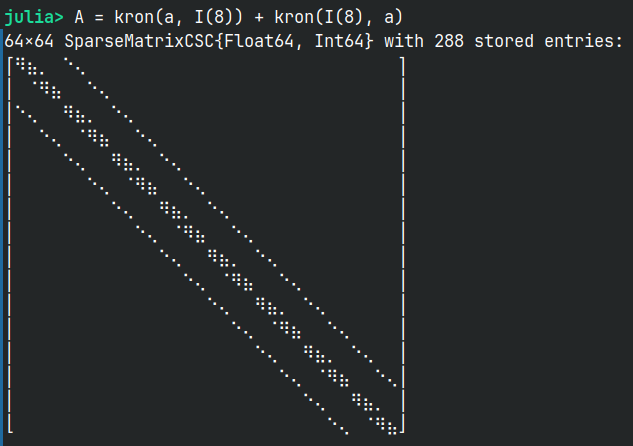# Help on how to describe a Matrix used for 2D Finite Differences using BlockBandedMatrices.jl

Some context

I’m dealing with the solution of a Poisson Equation with structure:

∇ψ[i, j] = -ω[i,j] => (∂²ψ/∂x² + ∂²ψ/∂y²) = -ω[i,j]


To represent the LHS, i’m using a Matrix that contains the coefficients used to calculate the second derivatives of ψ. Since i’m dealing with two dimensions, i’m using a Kronecker product to generate a n^2 x n^2 matrix for solving the case cited above, assuming that ψ and ω both have the size n x n. I reshape the ω matrix into a vector form, solve the linear system and then create the ψ matrix by reshaping it once again. The final form of the LHS Matrix A used to solve Aψ = -ω is something like:I purposefully reduced n to be equal to 8 to really show the sparsity pattern, as it becomes really hard to visualize on higher dimensions.

I currently am solving this system by pre-calculating the Cholesky/LDLT decomposition, because this linear system is a part of a bigger system of PDEs, and wrapped in a iterative algorithm, so it makes sense to cache it.

Here’s my actual question

I want to represent this final matrix form using a BlockBandedMatrix (or BandedBlockBandedMatrix in this case):

• Is that possible? It looks plausible to me, but i can’t understand how i would even begin to formulate it.
• If possible, would that representation bring some gains in performance? Currently, solving this linear system is the main bottleneck of my algorithm, and i tried some other methods like Iterative Solvers (CG and GMRES) or Multigrid Methods. Both fell short.

Would really appreciate some help. I love the Julia Community and the amount of available packages for use, but i really lack some basic understanding of some concepts that may seem obvious to the outside worldI’m skeptical, since the bandwidth for an n \times n grid will be 2n, so IIRC the complexity of a banded direct solve should be O(n^4) (rows × bandwidth²), whereas the complexity of a sparse-direct solve with SparseMatrixCSC should be O(n^3) (for a finite-difference matrix from an n \times n grid — see these slides, which are based on Tim Davis’ book).

1 Like

how big will n be for the real application?

Thx for the material. Will read into it. But you cited banded direct solving, would that change with a block banded matrix? Or it doesn’t matter in this case? I was hoping that the block structure would help represent the matrix by splitting the bigger bandwith in small blocks with smaller bandwiths. I haven’t had the time to study how the BlockBanded representation work behind under the hood, so maybe i’m missing something.

To be honest, i don’t know yet. The idea is to make is feasible for it to deal with higher orders of n. At the moment, biggest i’ve tried is 512. It can run, but with a big hit on performance.

The other day, i was trying to implement a distributed computing solution, but i don’t know how big n must be to see some gain in this case.

The first thing I would try would be to simply plug in some other sparse-direct solvers, e.g. Pardiso.jl or MUMPS.jl to see if they are faster for your problem; both of these can also support parallelism.

1 Like

I am trying out Pardiso.jl, but i haven’t found a way to properly cache the generated Factorization

So, great news. I managed to integrate PARDISO and also cache the factorization. Looks promising, thanks for the suggestion.

But i’ve read about how increasing the number of threads is not guaranteed to give you better performance (as in Autochecking BLAS Thread Settings · ThreadPinning.jl). Is this a thing for PARDISO? Currently, i’m defaulting setprocs! to define the same amount as in BLAS.get_num_threads()

That’s a thing for all parallel computing. Sometimes the cost of coordinating multiple threads/processes outweighs the computational gain, especially if the problem size is too small.

1 Like

I will do some tests and figure that out by experimentation then. Thank you so much for your help, i will be marking the thread as solved.